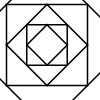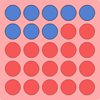#### You may also like### Tangram Tangle

If you split the square into these two pieces, it is possible to fit the pieces together again to make a new shape. How many new shapes can you make?### Shaping It

These pictures were made by starting with a square, finding the half-way point on each side and joining those points up. You could investigate your own starting shape.### All Change

There are three versions of this challenge. The idea is to change the colour of all the spots on the grid. Can you do it in fewer throws of the dice?

# Weighted Numbers

##### Age 5 to 7 Challenge Level:

Bethanni from McCabe School sent in a clear solution to the first part of the problem. She says:

If you have 3 on one side, and you have to balance it out, without using three 1s. You have to put on the other side an equal amount. That can only be a 2 and a 1.

Adele and Lottie from Aldermaston C of E Primary School answered the next parts of the question for us:

3 and 4 on one side need three 2s plus a 1 to make it balance.

They then went on to say:

These are the ways to make it balance with 10:
6+4
1+9
3+7
8+1+1
5+5
8+2
3+3+3+1
2+3+1+4
7+1+2
6+1+2+1
3+4+3
5+3+2
4+5+1
And lots more as long as they add up to 10. It doesn't matter how many weights you use, but they must add up to 10.

In the Spring of 2016 we had an email sent in from Oscar, Evie, Toby, Sophie, Theo and Godwin at Cranleigh Primary School:

We loved working on this problem this morning in our maths lesson. We found our answers by working systematically, from 1 all the way up to 10. We tried every possibility for numbers up to 10, starting like this:

1+1+1+1+1+1+1+1+1+1=10
2+1+1+1+1+1+1+1+1+1=10
2+2+1+1+1+1+1+1=10
2+2+2+1+1+1+1=10

We have found 14 ways so far and we can't wait to carry on!

That's great perhaps you'll send in more!  I like the way you have used a system so that you can be sure you find all the different ways of making 10.